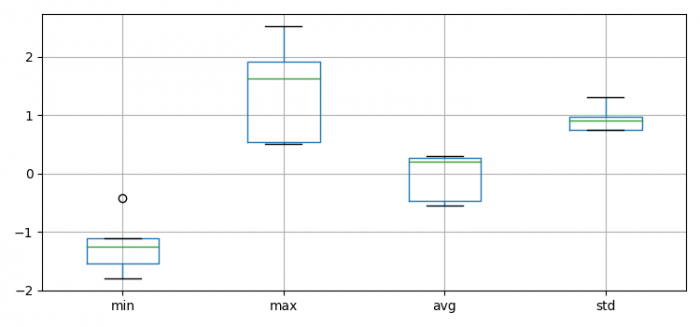# Box plot with min, max, average and standard deviation in Matplotlib

MatplotlibPythonData Visualization

To make a box plot for min, max, average and standard deviation in matplotlib,

## Steps

• Set the figure size and adjust the padding between and around the subplots.
• Create a random dataset of 5☓5 dimension.
• Find min, max, average and standard deviation from the data.
• Make a Pandas dataframe with Step 3, min, max, average and standard deviation data.
• Make a box plot from the dataframe column.

## Example

import numpy as np
import pandas as pd
from matplotlib import pyplot as plt
plt.rcParams["figure.figsize"] = [7.50, 3.50]
plt.rcParams["figure.autolayout"] = True
data = np.random.randn(5, 5)
min = data.min(0)
max = data.max(0)
avg = data.mean(0)
std = data.std(0)
df = pd.DataFrame(dict(min=min, max=max, avg=avg, std=std))
df.boxplot()
plt.show()

## Output